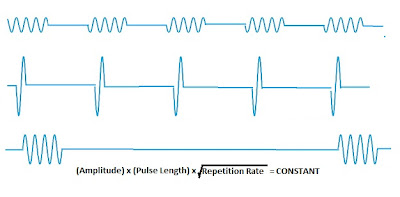## Monday, February 21, 2011

### What's the connection?

In my last posting, I showed that you could get a convert the formula for electromagnetic power density into a real-time picture of actual sine waves, but you got different results depending on how you chopped up the spectrum and how wide you chose the bandwidth. Here are three pictures of typical waveforms you can get from a given spectrum. The pictures are highly simplified to illustrate the most essential features of the waves. The single cycle of a sine wave is really intended to suggest hundreds or millions of cycles in general:It's not completely arbitrary. I've written in a formula at the bottom of the picture to show that the three waveforms must have a certain property in common. I've expressed this property as a mathematical formula because that's what people do, but the physical significance of this common property is what really matters. And it relates to ... the drunkard's walk!

If any of these wave trains are applied to a harmonic oscillator, they will tend to build up the vibration; but because the applied wave and the mechanical oscillator are in general out of phase with each other, the amplitude of oscillation will build up not steadily, but randomly. Like the drunkards walk.

What these three wave forms have in common is that if you apply them to a given oscillator over a period of time, they will cause the oscillation to grow at equal rates!

You don't even need to know much about the dynamics of a driven oscillator to see why this ought to be so. It goes back to the drunkard's walk. Let's compare the first two wave forms. The driving force in the second case is four times as great, applied over one quarter the time. That's why those two waveforms both give the same rate of growth.

The third case is interesting. Compared to the first waveform, it's the twice the impulse applied one quarter as often. It's like the drunkard who takes a giant two-meter step, but only once every four seconds. Figure it out ... he has the same rate of progress as the drunkard who takes regular one-meter steps every second.

So you can take your pick. Any one of these waveforms applied to a harmonic oscillator will cause it to grow at the same rate. What is the rate of growth? It appears to get slower and slower as the amplitude of oscillation builds up. But notice something funny. If we measure the size of the oscillation not by its amplitude, but by its total energy ... the growth rate becomes a constant. The energy of oscillation grows at a constant rate.

So why doesn't it build up without limit? Because as it oscillates, it becomes a transmitting antenna, and radiates outwards in all directions. There are formulas from antenna theory that will give us an exact number for this, but it's enough for now to undertand that the radiated power is proportional to the square of the amplitude. In other words, its proportional to the total power of the mechanical oscillator.

As the oscillator builds up, it emits power at a faster and faster rate. At some moment this becomes equal to the rate at which it is absorbing power. That is the point of equilibrium, and we can now calculate it.